## Tuesday, 26 October 2010

### Online maths help (a quiz with feedback)

If you are having problems with doing dilutions and concentrations calculations then you might like to do some practice using our online system, which can be found here on the School teaching website. Or enrol at Maths4Biosciences and take an online course.

The system can help with:

Percentage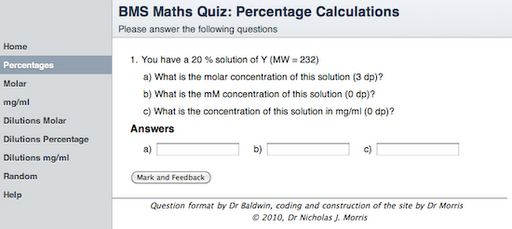Molarmg/ml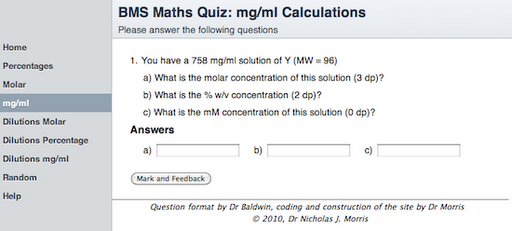Molar Dilutions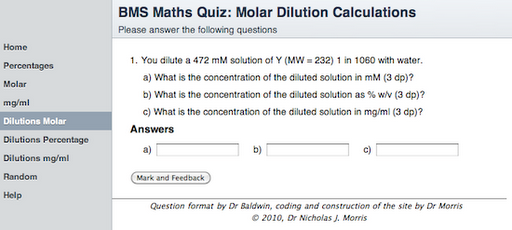Percentage Dilutionsmg/ml DilutionsHelp

The site also comes with additional help: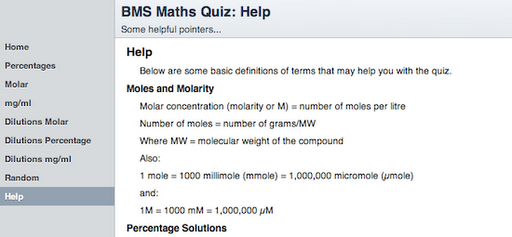Feedback

And if you get a question wrong, there is help in the feedback to explain how to do the calculation:## Wednesday, 20 October 2010

### Why can't I extrapolate the Bradford Assay graph if the Beer-Lambert Law applies?

If you are struggling with 'science maths' then have a look at Maths4Biosciences - there is also a course on Beer-Lambert Law and Spectrophotometry.

If you would like to test your skills working with the Beer-Lambert Law then you might like to look at the Spectrophotometry tests at: Maths4Biosciences.com.

One question I have been asked a number of times after the feedback on a practical is if the Beer-Lambert Law, A = ε . c . l, gives a straight line, then why can't I extrapolate the data to find the answer for one of my unknown proteins?

The Bradford Assay is an indirect (or colorimetric) method for estimating protein concentrations. The assay is actually based on a shift in the absorbance of a dye, Coomassie Brilliant Blue G-250, from 465 nm to 595 nm when it binds a protein under acidic conditions. That is, the Bradford assay is really measuring the amount of bound dye and NOT the amount of protein present (although the two are connected until the dye is all used). As the dye is limiting (i.e. you only put in a set amount), then you can reach a point when all the dye is bound, and no matter how much more protein you put in you will not see a change in absorbance. That is, you can increase the protein level to a point where available dye becomes exhausted so the graph would plateau.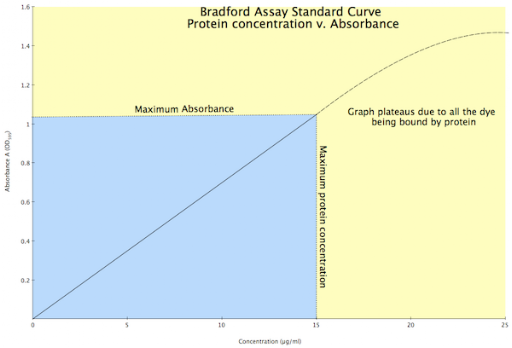Standard Curve for a Bradford Protein Assay

Any value that falls in the yellow area of the graph (i.e. a concentration of more than 15 µg/ml, or an absorbance greater than 1.02) cannot be used as the graph is no longer linear and is starting to plateau. Any absorbance that give a protein concentration below 15 µg/ml can be used as this is in the linear portion of the graph (i.e. blue area), and the Beer-Lambert Law applies as the dye is not limiting.

In the experiment you are working in the linear portion of the graph above (the blue area) and so the line is straight, i.e. it hasn't plateaued. Therefore we can say that between 0 and x µg/ml (or 15 µg/ml in the above) we have a certain absorption range, say 0 to y (where y is about 1.01 in the above figure). However, once we get above x µg/ml, or above the maximum absorbance y, we cannot say whether or not the graph has plateaued, so all we can say is the value is greater than x µg/ml. This may seem somewhat limiting (pardon the pun), but the Bradford assay can in fact detect protein concentrations over a 10-fold concentration range.

By the way, if we were measuring the protein directly, say at 280 nm, then we could apply the Beer-Lambert Law, in fact, if we knew extinction coefficient we wouldn't even have to do a standard curve.

If you are struggling with 'science maths' then have a look at Maths4Biosciences - there is also a course on Beer-Lambert Law and Spectrophotometry.

## Friday, 8 October 2010

### Help with printing pdfs.....

Some of the class have been having problems printing pdfs of my lectures if they have more than one slide per page. The following post will walk you through a solution to the problem.

If you are printing pdfs of lecture slides, at say 6 slides per page, on a Windows machine and they keep printing like this (or some variation of this):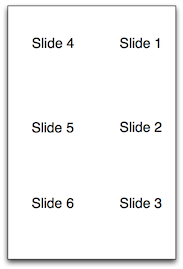Slides in the wrong order

Whereas you want them like this: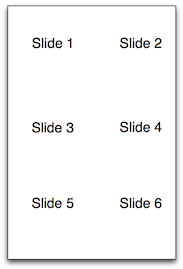Slides in the correct order

Then there is a problem with the way you have set up printing, that is, you have 'Auto rotate and scale' on (i.e. ticked).

To solve the problem follow these steps:

1. In the print dialog, make sure that 'Auto rotate and scale' is turned off (i.e. not ticked)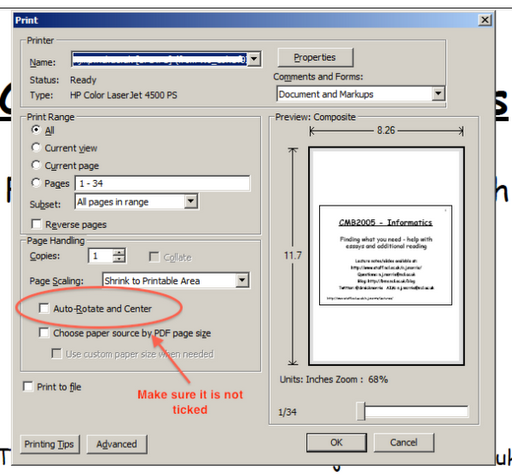Printer dialog screen

1. Click on Properties.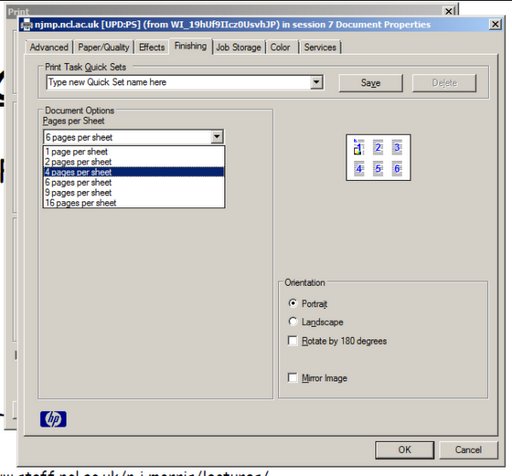Set the number of pages to print per page

1. Select (click) the 'Finishing' tab, and set the number of pages you want to print per page.
2. Set page orientation.
3. Click on OK to close the properties window.
4. Click on OK in the print dialog window to close it and to print the document.

## Wednesday, 6 October 2010

### The Beer-Lambert Law, a straight line and the units of the extinction coefficient

If you are struggling with 'science maths' then have a look at Maths4Biosciences - there is also a course on Beer-Lambert Law and Spectrophotometry.

If you would like to test your skills working with the Beer-Lambert Law then you might like to look at the Spectrophotometry tests at: Maths4Biosciences.com.

A number of the class seem to have had a problem in understanding the relationship between the Beer-Lambert Law and a straight line, and working out the units of the extinction coefficient (ε).

The Beer-Lambert Law states:

A = ε . c . l

Where:

A = absorbance
ε = extinction coefficient
c = concentration
l = path length (i.e. the distance the light travels through the sample)

So, what connection between this and a straight line, and what are the units of the extinction coefficient?

The units of the extinction coefficient

In my opinion the extinction coefficient has some of the craziest units out there.
Absorbance (A) has no units so the units of the extinction coefficient (ε) are determined by how the concentration (c) and path length (l) are being measured. That is, the units of the extinction coefficient must cancel out the units of the concentration and path length so that the absorbance can have no units!

A worked example.

A = ε . c . l

A = absorbance, units - so put in 1
ε = extinction coefficient, units - unknown
c = concentration, units - milli-Molar, mM
l = path length (i.e. the distance the light travels through the sample), units - cm

So...

A = ε . c . l

And, with units:

 = ε . [mM] . [cm]
Rearranging...
 / ε = [mM] . [cm]

ε = 1 / ([mM] . [cm])

or

ε = [mM]-1 . [cm]-1

So, the units are mM-1 . cm-1

This can be checked by putting it all back together:

A = ε . c . l

A = ([mM]-1 . [cm]-1) . [mM] . [cm]

This gives:

A = [mM]/[mM] . [cm]/[cm]

So, the mM and the cm cancel each other out, leaving no units for absorbance A.

A straight line

The Beer-Lambert Law:

A = ε . c . l

Where:

A = absorbance
ε = extinction coefficient
c = concentration, units
l = path length

The equation for a straight line is:

y = mx + c

Where:

c = the y intercept

If you plot concentration, against absorbance, then x = concentration and y = absorbance. Plus, from the Beer-Lambert Law we know that if concentration is zero, then absorbance must be zero.

A = ε . c . l
A = ε . 0 . l
A = 0
So...

y = mx + c
absorbance = m . concentration + c

From above, if concentration = 0, then absorbance = 0, hence c must be zero
y = mx + c
absorbance = m . concentration + c*
0 = m . 0 + c
c = 0

(* note, this c is the y intercept, and not the concentration)

Therefore...
y = mx + c
absorbance = m . concentration + 0
or
y = mx

Comparing:

y = mx
absorbance = m . concentration

With (and rearranging):

A = ε . c . l

A = (ε . l) . c

y = m . x
It can be seen that if y = absorbance, and x = concentration, then m (the gradient) must equal extinction coefficient (ε) multiplied by the path length, l, or ε . l. As l is typically 1 cm, then the gradient, m, must equal the extinction coefficient (ε).

If you are struggling with 'science maths' then have a look at Maths4Biosciences - there is also a course on Beer-Lambert Law and Spectrophotometry.